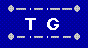# Analysis of two-color problems

There are two configurations:
• A single row, 1 x 6
• Two rows of 3, 2 x 3.

In all cases, we disregard rotations and reflections of the entire configuration.

For either configuration, there are four, which can be obtained by horizontal
or vertical flips, or the two together which is equivalent to a
180° rotation.

Two arrangements which only differ by permuting colors are not considered different either.

Let us give some names to the 6 tiles: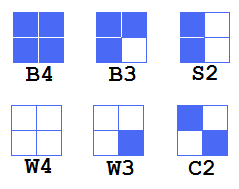• B4 (all blue)
• W4 (all white)

• B3 (3 blue, 1 white)
• W3 (3 white, 1 blue)

• S2 (Stripes)
• C2 (Checkerboard)

## 2 x 3

For the 2 x 3, there is a unique solution, which we can prove as follows.

Let's number the positions on the board this way:

We start by finding a place for the B4 piece.
By symmetry, positions 1, 3, 4 and 6 are equivalent
to each other, as are 2 and 5 (at least initially).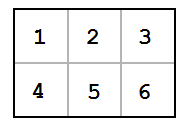So we have just two cases to consider: B4 in position 1 or B4 in position 2.

Taking position 2 first, we notice that since colors "bleed" across
edges and corners, all the places marked "b" will also be blue.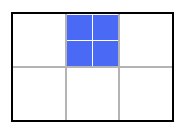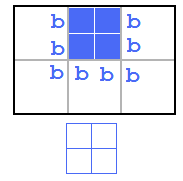That leaves no place for W4, the all white tile,
and so we see that starting with B4 in position 2

Therefore, B4 must go in position 1, bleeding as shown.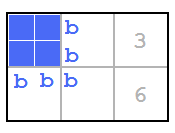The choices for W4 are now limited to places 3 and 6.

Trying position 6 first,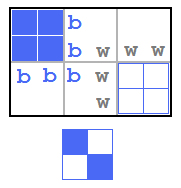we see there is no place for C2, the checkerboard,
since every empty position will have a solid colored edge.

That leaves position 3 for W4.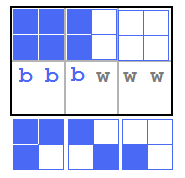Then C2 must go in position 5 (center, bottom row),
and that forces the placement of the remaining two pieces, B3 and W3 into place,
completing the solution.Here is the proof in tree form:## 1 x 6

The 1 x 6 has a total of 5 unique solutions, not counting reflections and rotations of the whole thing.

It is a bit more complicated, as it is somewhat less constrained.

First we switch to a new color scheme: Blue and Red instead of Blue and White.
That means changing two of the tile names to R4 and R3 (from W4 and W3).

The board and tiles now look like this: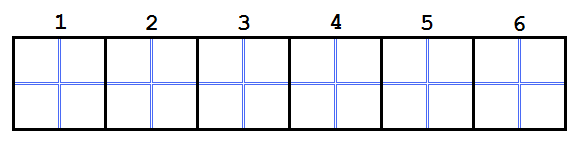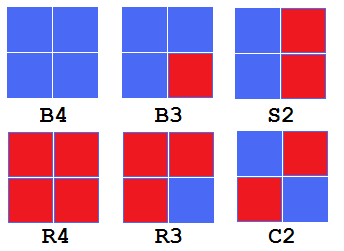Again we have 4 symmetries, as we did with the 2 x 3.

Symmetrical positions are in pairs:
1 2 3 with 6 5 4 respectively.

We begin with the positions of B4 and R4.

There (6 choose 2) = 15 ways to place them in a row of 6.

First we eliminate the 5 positions where they are adjacent: 1-2, 2-3, 3-4, 4-5, 5-6.

Then we have 1-3, 1-4, 1-5, 1-6.

2-4, 2-5, but (2-6 = 1-5).

3-5 (=2-4) and 3-6 (=1-4) are not new.

4-6 (=1-3) is not new either. Summing up, we have:

• 1 and 3 ( 1 solution )
• 1 and 4 ( 0 )
• 1 and 5 ( 1 )
• 1 and 6 ( 1 )
• 2 and 4 ( 2 )
• 2 and 5 ( 0 )

Let's take these one by one:

### 1 and 3: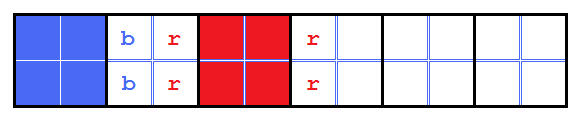S2 must go in position 2.

Then, C2 cannot go in position 4, which leaves just:

• #### C2 in position 5 (1 solution)

In this case R3 must then go in position 4, leaving just B3 in position 6.It might seem that rotating C2 by 90° and then rotating
R3 and B3 accordingly would change the solution, but that
is equivalent to a vertical flip of the whole thing.

• #### C2 in position 6 (0 solutions)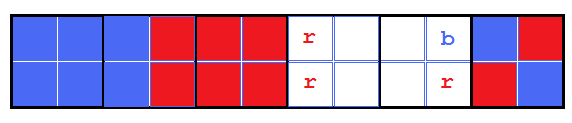B3 cannot go in position 4, so it must go in 5. Then remaining space calls for S2, but all we have is R3.

### 1 and 4: (0 solutions)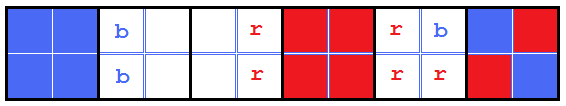R3 must go in 5.
The remaining spaces are 2 and 3, and remaining tiles are S2 and B3.

No matter which position S2 is placed in, it will require another solid color tile in the last place, and both have been used.

### 1 and 5 (1 solution)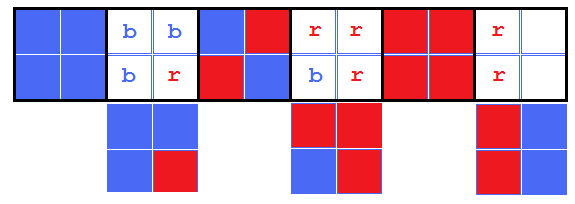C2 cannot go in positions 2, 4, or 6, so it must go in 3.

Positions 2 and 4 must then be filled by B3 and R3,
leaving just S2 which successfully fills position 6.

### 1 and 6: (1 solution)

C2 can only go in 3 and 4, and it doesn't matter which, due to symmetry, so let's say it's 4.Again, R3 must go in position 5.

We have left positions 2 and 3 and tiles B3 and S2.
If we put S2 in position 2, we need another R3.
So we must put S2 in position 3 (horizontal stripes), and then B3 fits nicely.

It is interesting to note that this is the only possible way to get the S2 stripes horizontal.

That is because, unless both B4 and R4 are on the ends,
they each need solid-color edges on both sides,
and the only way to get that is to use B3 (or R3) on
one side, and S2 with vertical stripes on the other.

### 2 and 4: (2 solutions)Positions 1, 3, and 5 all will have at least one solid-color edge, forcing C2 into position 6.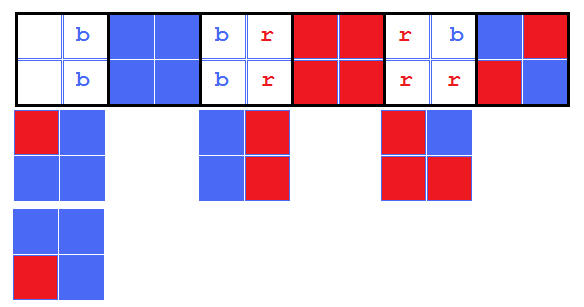That forces R3 into position 5.

S2 is forced to position 3, leaving B3 for position 1.

However, it can be placed in either of two rotations,
since the red quadrant is on the "outside".

We could also rotate C2 on the other end,
forcing a rotation of R3. But that does not change the overall
picture, merely interchanging the two rotations of B3.

No matter how its done, two outside corners are red, two are blue,
and either they match up (both red top, or both red bottom),
or they don't (blue-red on top, red-blue on bottom, or the reverse),
and that is what makes for two solutions, but not more.

### 2 and 5: (0 solutions)Every open square now will have a solid-color edge (vertically), leaving no place to put C2.

## All the solutions

In this figure we present all the solutions.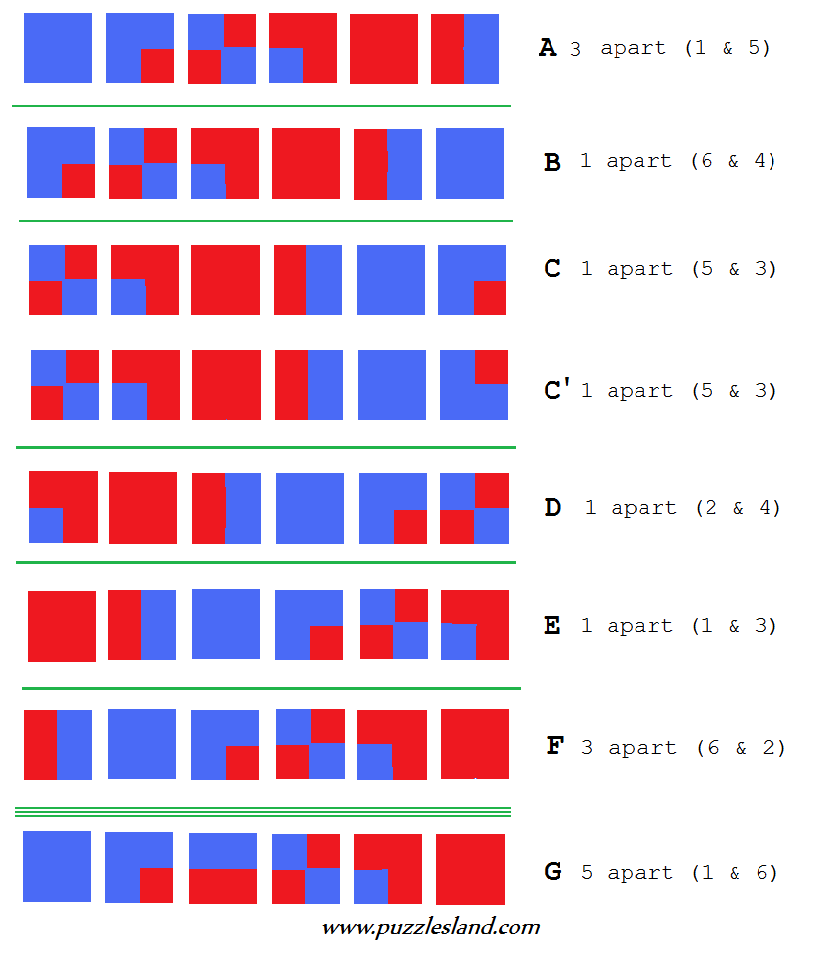The figure is derived by taking solution A, and cycling the tiles.
In each case (through F), the leftmost tile is removed and replaced at the far right,
then the line is moved one space to the left.
C' differs from C by rotation the B3 tile at the far right.

G is added separately since a rotation of it would not be a valid solution:
the two solid-color tiles would be adjacent.

But wait, there are eight here, not 5, what gives?

There are three pairs of equivalent solutions:

• A & F are horizontal flips of each other, with the colors swapped.
• B & E are similarly related.
• C & D are also a similar pair.
The 5 solutions are then: A, B, C, C' and G;